Courses

# Change in Profit-Sharing Ratio Among the Existing Partners (Part -1) Commerce Notes | EduRev

## TS Grewal Solutions - Class 12 Accountancy

Created by: Nipun Tuteja

## Commerce : Change in Profit-Sharing Ratio Among the Existing Partners (Part -1) Commerce Notes | EduRev

The document Change in Profit-Sharing Ratio Among the Existing Partners (Part -1) Commerce Notes | EduRev is a part of the Commerce Course TS Grewal Solutions - Class 12 Accountancy.
All you need of Commerce at this link: Commerce

Page No 4.37:

Question 1: A and B are sharing profits and losses equally. With effect from 1st April, 2019, they agree to share profits in the ratio of 4 : 3. Calculate individual partner's gain or sacrifice due to the change in ratio.

Old Ratio (A and B) = 1 : 1

New Ratio (A and B) = 4 : 3

Sacrificing (or Gaining) Ratio = Old Ratio − New Ratio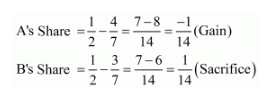∴ A’s Gain = 1/14

B’s Sacrifice = 1/14

Page No 4.37:

Question 2: X, Y and Z are sharing profits and losses in the ratio of 5 : 3 : 2. With effect from 1st April, 2019, they decide to share profits and losses in the ratio of 5 : 2 : 3. Calculate each partner's gain or sacrifice due to the change in ratio.

Old Ratio (X, Y and Z) = 5 : 3 : 2

New Ratio (X, Y and Z) = 5 : 2 : 3

Sacrificing (or Gaining) Ratio = Old Ratio − New Ratio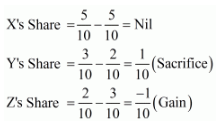∴ Z’s Gain = 1/10

Y’s Sacrifice = 1/10

Page No 4.37:

Question 3: X, Y and Z are sharing profits and losses in the ratio of 5 : 3 : 2. With effect from 1st April, 2019, they decide to share profits and losses equally. Calculate each partner's gain or sacrifice due to the change in ratio.

Old Ratio (X, Y and Z) = 5 : 3 : 2

New Ratio (X, Y and Z) = 1 : 1 : 1

Sacrificing (or Gaining) Ratio = Old Ratio − New Ratio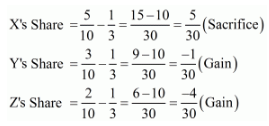∴ Y’s Gain = 1/30

Z’s Gain = 4/30

X’s Sacrifice = 5/30

Page No 4.37:

Question 4: A, B and C are partners sharing profits and losses in the ratio of 5 : 4 : 1. Calculate new profit-sharing ratio, sacrificing ratio and gaining ratio in each of the following cases:
Case 1. C acquires 1/5th share from A.
Case 2. C acquires 1/5th share equally form A and B.
Case 3. A, B and C will share future profits and losses equally.
Case 4. C acquires 1/10th share of A and 1/2 share of B.

Calculation of New Profit Sharing Ratio
Case 1:

A:B:C=5:4:1(Old Ratio)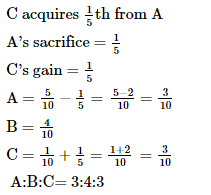Case 2: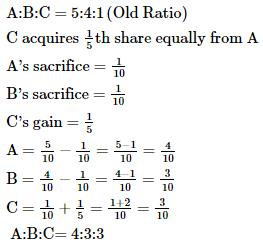Case 3: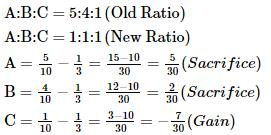Case 4: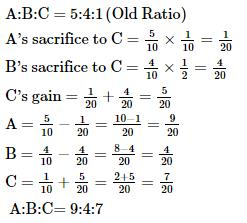Page No 4.37:

Question 5: A, B and C shared profits and losses in the ratio of 3 : 2 : 1 respectively. With effect from 1st April, 2019, they agreed to share profits equally. The goodwill of the firm was valued at ₹ 18,000. Pass necessary Journal entries when: (a) Goodwill is adjusted through Partners' Capital Accounts; and (b) Goodwill is raised and written off.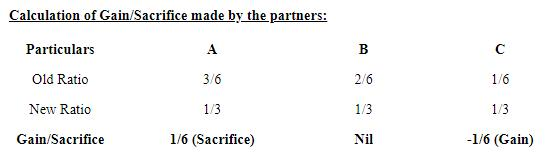Case a)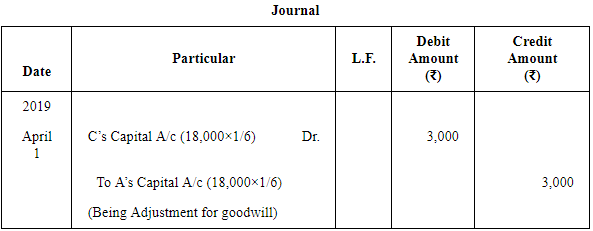Case b)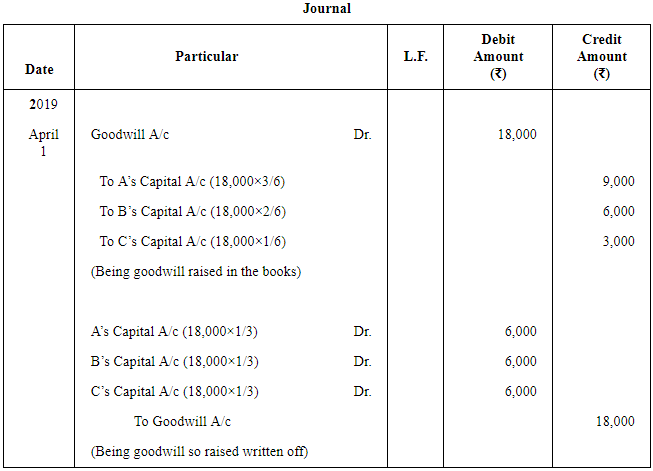Page No 4.38:

Question 6: X, Y and Z are partners sharing profits and losses in the ratio of 5 : 3 : 2. From 1st April, 2018, they decided to share profits and losses equally. The Partnership Deed provides that in the event of any change in the profit-sharing ratio, the goodwill should be valued at two years' purchase of the average profit of the preceding five years. The profits and losses of the preceding years ended 31st March, are: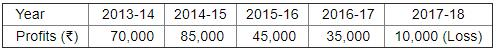You are required to calculate goodwill and pass journal entry.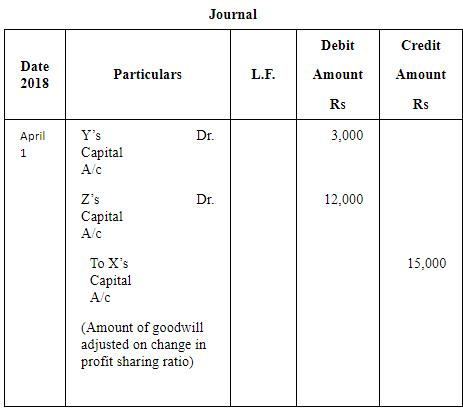Working Notes:

WN 1 Calculation of Sacrificing (or Gaining) Ratio

Old Ratio (X, Y and Z) = 5 : 3 : 2

New Ratio (X, Y and Z) = 1 : 1 : 1

Sacrificing (or Gaining) Ratio = Old Ratio − New Ratio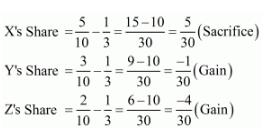WN 2 Calculation of Goodwill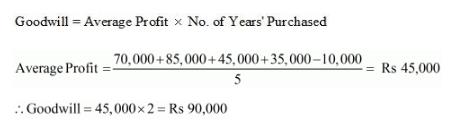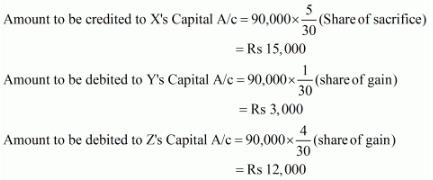Page No 4.38:

Question 7: Mandeep, Vinod and Abbas are partners sharing profits and losses in the ratio of 3 : 2 : 1. From 1st April, 2019 they decided to share profits equally. The Partnership Deed provides that in the event of any change in profit-sharing ratio, goodwill shall be valued at three years' purchase of average profit of last five years. The profits and losses of past five years are:
Profit − Year ended 31st March, 2015 − ₹ 1,00,000; 2016 − ₹ 1,50,000; 2018 − ₹ 2,00,000; 2019 − ₹ 2,00,000.
Loss − Year ended 31st March, 2017 − ₹ 50,000.
Pass the Journal entry showing the working.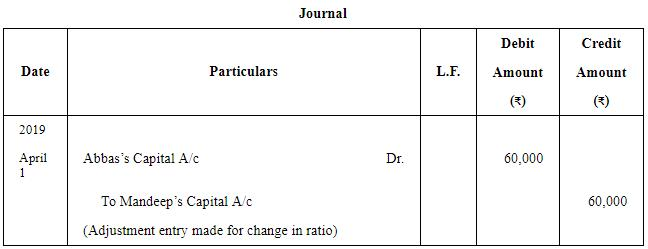Working Notes:

WN1: Calculation of Sacrifice or Gain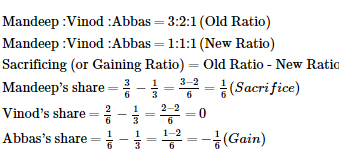WN2: Valuation of Goodwill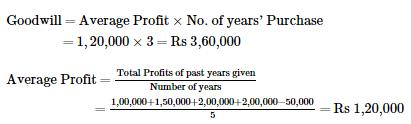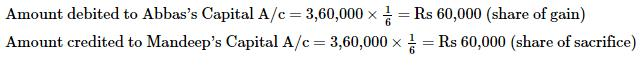Page No 4.38:

Question 8: X, Y and Z are partners sharing profits and losses in the ratio of 5 : 3 : 2, decided to share future profits and losses equally with effect from 1st April, 2019. On that date, the goodwill appeared in the books at ₹ 12,000. But it was revalued at ₹ 30,000. Pass Journal entries assuming that goodwill will not appear in the books of account.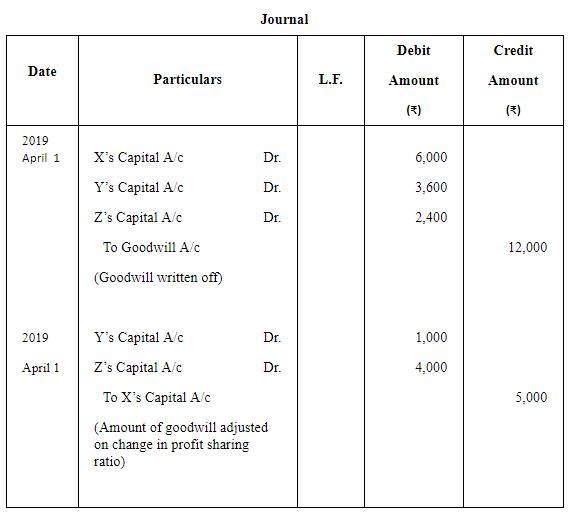Working Notes:

WN 1 Calculation of Sacrificing (or Gaining) Ratio

Old Ratio (X, Y and Z) = 5 : 3 : 2

New Ratio (X, Y and Z) = 1 : 1 : 1

Sacrificing (or Gaining) Ratio = Old Ratio − New Ratio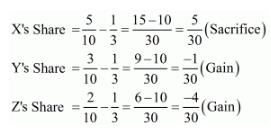WN 2 Writing off of Old Goodwill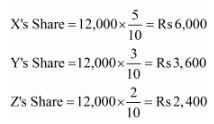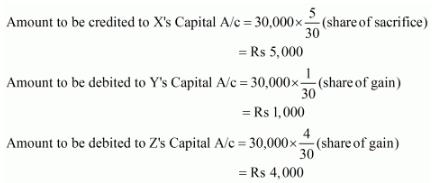Page No 4.38:

Question 9: A and B are partners in a firm sharing profits in the ratio of 2 : 1. They decided with effect from 1st April, 2018, that they would share profits in the ratio of 3 : 2. But, this decision was taken after the profit for the year ended 31st March, 2019 of ₹ 90,000 was distributed in the old ratio.

The profits for the year ended 31st March, 2017 and 2018 were ₹ 60,000 and ₹ 75,000 respectively. It was decided that Goodwill Account will not be opened in the books of the firm and necessary adjustment be made through Capital Accounts which on 31st March, 2019 stood at ₹ 1,50,000 for A and ₹ 90,000 for B.
Pass necessary Journal entries and prepare Capital Accounts.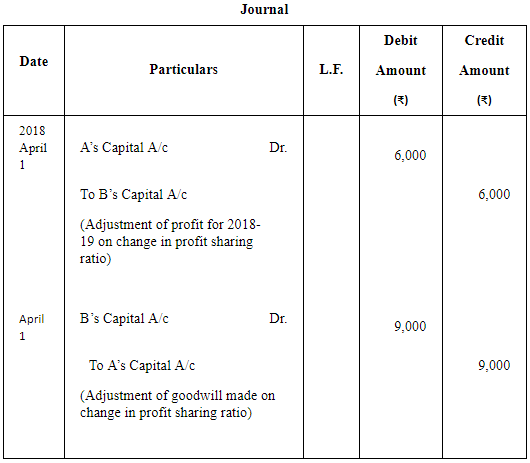Partners’ Capital Accounts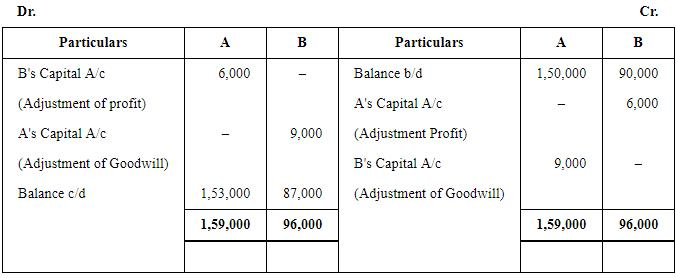Working Notes:

WN 1 Calculation of Sacrificing (or Gaining) Ratio

Old Ratio (A and B) = 2 : 1

New Ratio (A and B) = 3 : 2

Sacrificing (or Gaining) Ratio = Old Ratio − New Ratio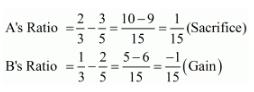WN 2 Adjustment of Profit for 2016-17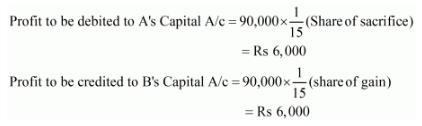WN 3 Calculation of New Goodwill
Goodwill=Profit of 2014−15 +Profit of 2015−16
=60,000+75,000=Rs 1,35,000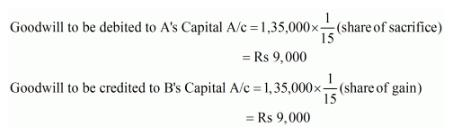Page No 4.38:

Question 10: Jai and Raj are partners sharing profits in the ratio of 3 : 2. With effect from 1st April, 2019, they decided to share profits equally. Goodwill appeared in the books at ₹ 25,000. As on 1st April, 2019, it was valued at ₹ 1,00,000. They decided to carry goodwill in the books of the firm.

Pass the Journal entry giving effect to the above.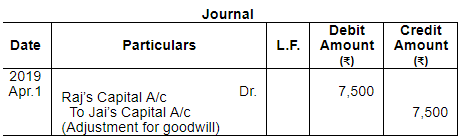Working Notes:
Calculation of Gaining/Sacrificing Ratio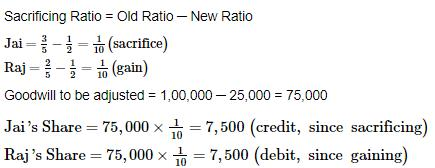41 docs

,

,

,

,

,

,

,

,

,

,

,

,

,

,

,

,

,

,

,

,

,

;